# Team:ULB-Brussels/Modeling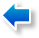Bacteria planned death system Extra-Scientific issues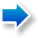Modelisation

1. Hydrogen module's modelisation

Questions from the Wetlab part

Questions asked by the dihydrogen module team were general: how much would the deletion of the whole, or part, of the proposed genes increase the amount of dihydrogen produced? To answer these questions the metabolic pathway of mixed acid fermentation had to be modeled before we could, after elimination of equations for part of the pathway, observe the increase in term of dihydrogen production.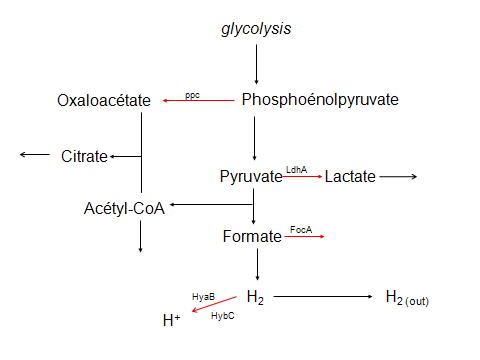Figure 1. In red, reactions catalyzed by enzymes whose deactivation should increase H2 production; the gene name corresponding to the enzyme is put on top of the arrow. We didn't include all the genetic regulations in that figure, as they are not relevant to our modeling effort.

Initial equations and further enhancements

We distinguish two types of creation or destruction terms. Linear terms are used when a reaction is assumed spontaneous and non-catalyzed. They are of the coefficient.reagent concentration type. Then the big category of non-linear terms, used in more complex situations where enzymes catalyze the reaction. In the H2 module, these non-linear terms are Michaëlis-Menten like, which is the typical kinetic of enzyme-catalyzed reactions. In the next section, we will talk about other non-linear terms, used when we model systems with important genetic regulations. Here are, the first equations which represent the metabolic pathway of the mixed acid fermentation.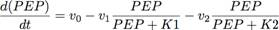v0 is a constant of production of the phosphoenolpyruvate by glycolysis. The two next terms are enzymatic terms of first order, characterizing the catalyzed reaction ADP + PEP <=> ATP + PYR + 2H+ (v1) and the catalyzed reaction PEP + CO2 + H2O <=> OXA + P + H+ (v2)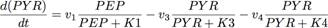The first term is the production of pyruvate, from phosphoenolpyruvate The second term (v3) is a term of Michaëlis-Menten for the catalyzed reaction PYR+ CoA <=> FOR + AcCO. The third term (v4) is once again a non-linear term representing the catalyzation of pyruvate in lactate, following the reaction NADH + PYR + H+ <=> LAC + NAD+ )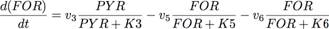The first term is the formation of the formate, from pyruvate. The second term (v5) represents the catalysation of FOR + H+ <=> CO2 + H2. The third term (v6) represents facilitated diffusion of formate, by specific and non-specific permeases. It's again an enzymatic reaction, with a typical non-linear kinetic.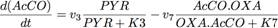The first term is the formation of acetyl-CoA from pyruvate. The second (v7) is a non-linear term of the second order in OXA and AcCO, representing the reaction OXA + AcCO+ H2O <=> CIT + CoA + H+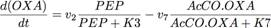The first term is the apparition of oxaloacetate from PEP The second term is a non-linear term of the second order for OXA and AcCO, representing the reaction OXA + AcCO+ H2O <=> CIT + CoA + H+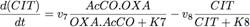The first term is the production of citrate from acetyl-CoA and oxaloacétate The second term is the consumption of citrate, in the following reaction.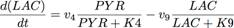The first term is the production of lactate from pyruvate. The second term (v9) is the facilitated diffusion term for lactate.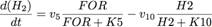The first term is the production of H2 from formate. The second term is the of H2, for example by hydrogenases

We then realized the importance of an equilibrium term in the last reaction leading to the formation of hydrogen  (FOR + H+  <=> CO2 + H2.),this product can accumulate, which encourages the reverse reaction.
We also refined the kinetic of the reaction OXA + AcCO+ H2O <=> CIT + CoA + H+, using a non-linear term which considers the concentration and Michaëlis-Menten constant specific of each substrate.
Finally, and to more easily observe the benefit of each deletion, we added an equation modeling the output speed of H2 diffusing out of the bacteria.
Here are the final equations :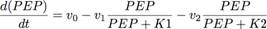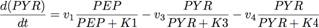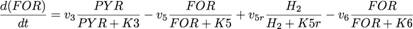Where the term in v5r is the equilibrium term characterizing the reverse reaction of the H2 production. This term has been added to account for the balance of the reaction, and avoid an irrealist accumulation of H2 in the cell.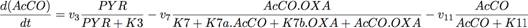Where the term (v7) is a modification of the preceding term in v7, modeling a more general kinetic, to better take into account the differences between the two substrates. Where the second term (K7b) is a term of utilization of acetyl-CoA in later reactions.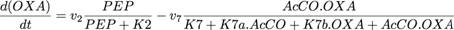With the same modification than above.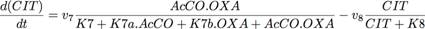With the same modification than above.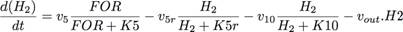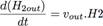Where the term in v5r is the equilibrium term characterizing the reverse reaction of the H2 production. This term has been added to account for the balance of the reaction, and avoid an irrealist of H2 in the cell.

Results

Such a differential equation system can't be analytically solved. We had to use a specialized software able to numerically integrate the system. To do that, we used the XPP software, specifically designed to model biological systems. This software allows us to study the evolution of each variable depending on time. It also has an extension, Auto, able to draw stability diagrams of the systems. At first, we only vary the parameters, depending on the expected wetlab results. For example, v2=0 means the PPC gene has been successfully Knocked Out (see table 1 for the corresponding between genes deletions and the parameters.). We can see, in the diagram 1, the theoretical effects of such a deletion on the intra-cellular concentration of dihydrogen at the steady-state: it's almost doubled (from 0.057 to 0.118). In the same way, we put each parameters to a nul value, alternately and in a combined way, to observe the effects of the deletions and their optimal combination.

 Genes to be desactivated Corresponding parameters Ppc v2 LdhA v4 FocA v6 HyaB et HybC v10

Table 1. Correspondance between experimentally Knock-Out genes and the parameters to be set at null value.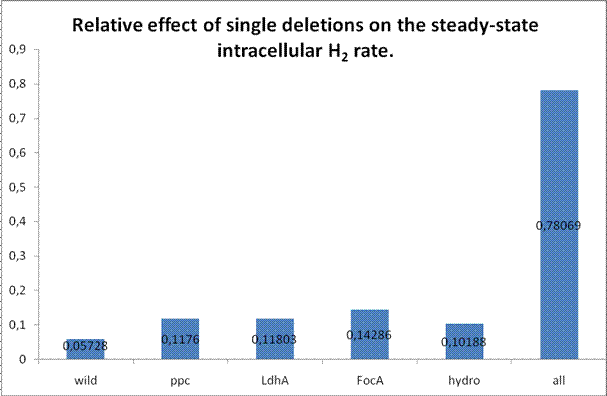Diagram 1. Only one deletion in the mixed acid fermentation pathway can double its output. We can observe the major effect of the FocA deletion.

We noticed that for double deletions, the results cluster in three increased levels of production (cf. Diagram 2). A deletion with particularly strong associated effect: FocA. LdhA and PPC are middle effect deletions, whereas the deletion of both hydrogenases seems to have a lesser effect on productivity. Indeed, the combinations including FocA are always in the two higher levels of production, while those including the hydrogenases are always in the two lowest levels.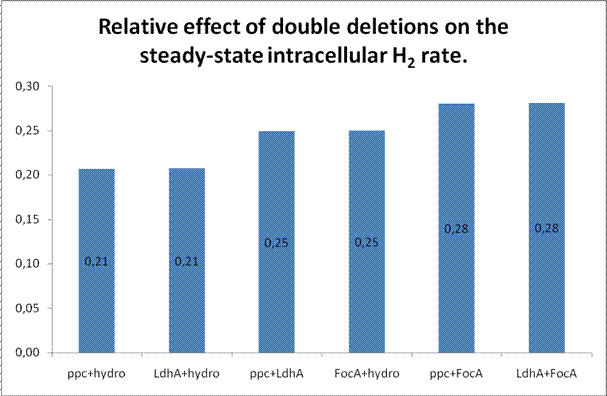Diagram 2. We immediately notice the clustering in three levels of production, with the double deletion including FocA having a much greater influence on the dihydrogen rate than those including the both hydrogenases.

In triple deletions, the above results are confirmed and show even more the importance of FocA in dihydrogen production, while the weakness of the hydrogenases deletion seems less important. Indeed, we notice here two very marked dihydrogen production levels, one being for bacteria missing FocA and two other genes (no matter which) and the other one for bacteria with a functional FocA.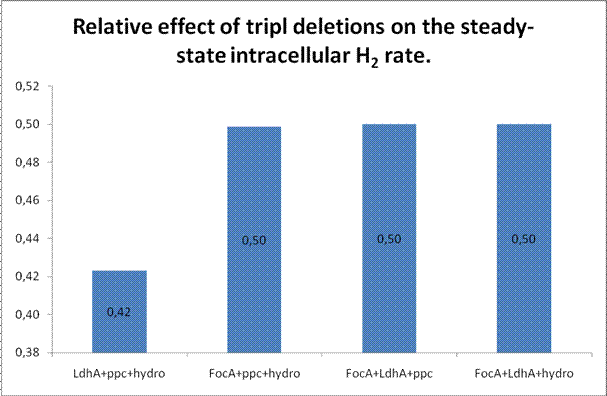Diagram 3.FocA deletion seems prevalent in the effect of the dihydrogen production, whereas the hydrogenases seem poor targets for the deletion. We can observe that the triple deletions increase dihydrogen production up to 9 times compared to the wild type bacteria.

There is an important clarification to make here. The intracellular concentration in dihydrogen represented here are in no case absolute values, they are given in arbitrary units. Generally speaking, all of the numbers presented in this section are only for a comparative purpose. This is easily understood if we remember the largely empirical way to establish the parameters of the differential equations.

1. Cell death module's modelisation

Questions from the Wetlab part

Here, after an initial modeling to theoretically validate the chosen system, we had to answer more specific questions, and with an immediate application : it was to choose the relative and absolute strength for the Ribosome Binding Sites (RBS) upstream of the genes used in this module, and to model the possible influence of the sequence the genes are built into. To obtain a functioning module, we wanted the system created by the interactions of both plasmids not to compromise the long-term population density. And, in the event of a tenfold dilution, for the density of bacteria to immediately fall with no new bacteria growth, this would model the escape of our modified bacteria in the river.

Initial equations and further enhancements.

When we have, like in this model, a certain complexity in the genetic regulations and we do model them, we generally use Hill terms, in addition to Michaëlis-Menten terms. Hill terms are non-linear, directly derived from Michaëlis-Menten like laws. They are proportional to C^n/C^n+K^n with n>=1 and where C is the concentration of the reactive and K in a constant
To determine which order of the Hill terms to choose, we used Hill term order equals the number of effective regulations levels. This is because we see at each regulation level, proteins characterized by a Michaëlis-Menten type kinetic. If there are two regulations levels, we can reasonably assume that the saturation effects combine.
In a simple model, like the one of the dihydrogen, we can approximate that the amount of transcribed genes equals the amount of traduced protein, thus the order equal one.
It's interesting to note that the order of the Hill's term is representative of the complexity degree of the dynamics of a system; also, terms with an order equal or superior to two often have complex stability diagrams, including bistability phenomenon..
This model is richer in regulations level, since we consider the details of genetic expression and its regulation. We cannot posit anymore that the amount of ARN is constant, and we have to take into account this variability of transcription, the complexity of this ARN stage, with the cooperative effects of the ARN-polymerase complex, we attribute a second order to the Hill terms put for the creation and consumption steps of the proteins we model. The first equation is more specific, since it models the bacterial density. This density classically increase, with an exponential phase followed by saturation of the system, hence the non-linear term of the logarithmic growth. The population, in addition to that density-limited growth, is also killed by the poison, proportionally to the bacterial density.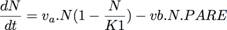The first term is the natural growth tendency of a bacterial population.
The second term is the cell death term due to the poison.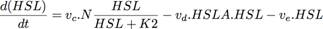The first term express the fact that the signal-molecule HSL (cf. the Cell death module in Wetlab part, for a more detailed introduction to the role of signal molecules and a characterization of HSL) induce his own expression (it's here a typical induction, which obeys, too, to the saturability of substrates law), and that this is modulated by the bacterial density: the more bacteria we have, the more probable will be the entry of HSL in the cell. The second term is a degradation term of HSL by the specific enzyme; the third one is a non-specific degradation or diffusion term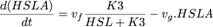The first term express the inhibition by HSL of the promoter which controls the gene coding for the HSLase.
The second term is a non-specific degradation term.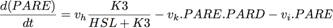The first term is identical to the one of the above equation, although with a different coefficient, because of the possibility of a different strength RBS. The second term expresses the mutual neutralization of the antidote and poison, by complex formation. The third term is a non-specific degradation term.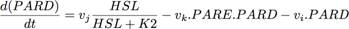The first term express the activation by HSL of the promoter, just as in the HSL equation but with a different coefficient due to RBS.

In a second time, to refine the system, we also chose to better take into account the subtleties of the culture medium, in which we add HSL in growth conditions, by clearly separating the two co-activators (or inhibitors) of the promoter. It was also to add equations, permitting to better take into account the formation of complexes, important in this system, since these complexes activate or inhibit the whole system. We again explicitly made the difference between the concentration in ARN and in protein.
Finally, we have distinguished the intra and extra-cellular HSL, to better take into account the HSL massive outflow phenomenon, by diffusion, during the dilution. This will allow our model to predict a possible peak of HSL, causing a partial stability (and even a slight initial growth) of the population, immediately after the dilution.
Here are the final equations :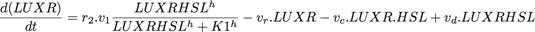LUXR is at the same time the transcript of the gene and the corresponding protein: the coactivator (or inhibitor) with HSL of the promoters. R2 is a coefficient put to model the relative strength of the RBS. LUXR is under control of the promoter activated by the LUXR-HSL complex, hence the creation term, and we notice the second order of the Hill term. The second term is a non-specific degradation term and the last one comes from the dissociation of the complex.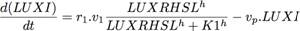LUXI designates the gene coding for HSL. We notice again the coefficient of the RBS and the activation by the complex, together with the non-specific degradation term.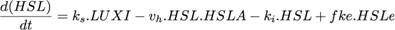HSL is translated using the LUXI transcript, hence the first term. The second one is a degradation term by the HSLase, proportional to this enzyme and to HSL. The third term is a loss term due to diffusion outside of the cell and the last one symbolizes the reverse phenomenon: the entry of extra-cellular HSL. Fke is a function of N, it allows us to model that, the more dilute the population is, the more HSL will leave the cells by passive diffusion. This will explain the massive outflow of HSL observed at the very first stages following an important dilution.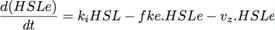HSLe (extra-cellular) is modulated by the diffusion in and out of bacteria. The last term is a loss term: when HSLe is too far away, it has no influence on the system anymore.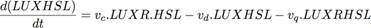LUXRHSL represents the complex formed by these two signal molecules. Its concentration is proportional to the one of its constitutive parts, minus the quantity of complex that dissociates and a non-specific loss term.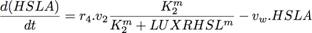Once again, we can see a coefficient put for the RBS. Here, the complex formed by the signal molecules inhibits the activity of the HSLase, which is under control of the poison-promoter. There is also a non-specific degradation term. .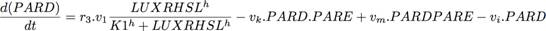PARD is the antidote, thus under control of the promoter activated by the signal-complex, hence the first term. The next one is due to the complex formation of PARD with the poison, and the third one to the dissociation of the complex. The last term is of non-specific loss.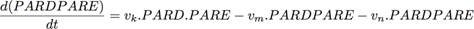The antidote-poison complex is formed from these two molecules and degraded by their dissociation. There is also a non-specific degradation term.

Results.

Before we tried to answer the questions asked by the wetlab part, we tried to establish the different values of the parameters (and the order of the Hill's terms) allowing a satisfying modeling of the module, and thus mathematically validating the chosen system. These results will be introduced at the end of this section, because they are a summary of the modeled behavior of the bacterial population, but we have to keep in mind that this stage is the very first one.
The first question answered a practical interest, because the main goal was to model the ideal relative value of RBS for each genes carried by plasmids. So we first simulated various configurations in this way.
We managed to vary the values of coefficients used in the above equations, which symbolized coded proteins on genes.
We studied the behavior of our population before and after a ten-order dilution as we were varying the RBS relative values (ones compared to each other). All parameters that gave an unstable population in the reactor or, to the contrary, stable or increasing population after dilution were immediately rejected.

Here are the main results obtained during our simulations:

Note that:
- According to the equations r1, r2, r3 represent the strength of "antidote" RBS and r4, r5 represent the strength of "poison" RBS.
- r1 represents LUXI RBS, r2 represents LUXR RBS, r3 represents PARD RBS, r4 represents HSLase RBS, r5 represents PARE RBS.
- We estimate that 6 units of time on the graphics represent 1 hour. We obtained this estimation by looking at the time a population needs to stabilize itself.

Step 1:
Vary R=r1=r2=r3=r4=r5

In the reactor:
For small values of R (<1 "relatively") the population collapses drastically after 5 or 6 hours spent in the reactor.
For higher values of R (>1) the population stabilizes itself. The bigger the value, the higher the population stabilizes itself.

In the river:
For small values of R (<1) the population is stable, which is a situation to absolutely avoid.
For higher values of R, we first observe a "baby-boom" quickly followed by a fall of population. (The importance of both of these phenomenons is proportional to R.)

A first result is obvious: we will have to work with powerful RBS.

Step 2:
Vary the poison (r4 = r5) and the antidote (r1 = r2 = r3) separately.
According to previous results, we will only use high values of RBS. (>1 "relatively")

Step 2.1 Improve the poison/antidote ratio

Results in the reactor:
- For a ratio of 1.05 (poison 5% stronger than antidote): during the 24 first hours, we only loose 5% of the population (compare to a ratio of 1), but later, the population does not stabilize itself.
- For a ratio of 1.1 (poison 10% stronger than antidote): during the 24 first hours, we loose 15% of the population (compare to a ratio of 1), and after 24h the population collapses.
- For a bigger ratio, the population collapses too quickly.
cf picture 1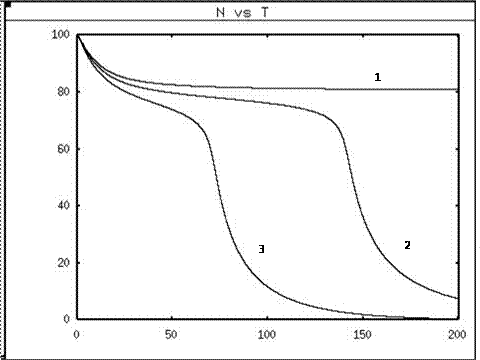Picture 1: Evolution of the population density in the reactor for different values of the ratio poison/antidote: 1,05 ; 1,1 and 1,3. Respectively curves 1, 2 and 3.

Results in the river:
The population falls down more quickly when the ratio increases, but the difference is not that interesting.

Step 2.2 Improve the antidote/poison ratio

Results in the reactor:
- Increasing the ratio until 1.5 enables the population to reach a stable state at 92% of its initial situation. Compare to 85% for a ratio of 1. The bigger the ratio, the higher the population will stabilize itself.

Results in the river:
- If the ratio is over 1.5 the population will not decrease any more.
- It is better that we do not go over a ratio of 1.2 in order to avoid a huge baby-boom (inevitable if the ratio is bigger than 1).

Conclusion for step 2:

In the reactor:

If we increase the poison by 10% (max), it does not have significant effect while t<24h.
If we increase the antidote by 20%, the population stabilizes itself at 90% of its initial situation. Compare to 85% for a ratio of 1 . As shown on picture 2.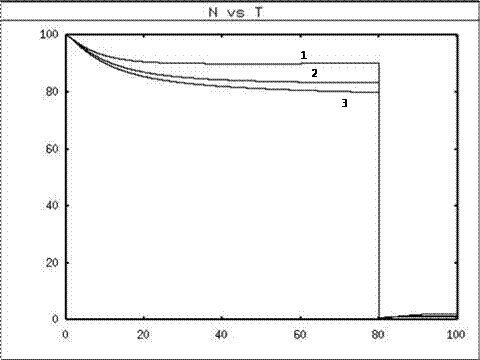Picture 2: evolution of the population density for 3 different values of the ratio antidote/poison : 0,9 ; 1 and 1.2. Respectively curves 1, 2, 3. N=population density and T=time.

In the river:
If we stand in a bracket of +10% poison/+20% antidote, our population collapses in a significant way.

Step 3 Vary antidote ones compare to each other, and similarly for poisons.

If we keep in mind the last results (rules) we obtained (mainly, r>1 and the fact that ratio antidote/poison stands between 0.9 and 1.2),
the effects on the population are not that different from the previous cases, whatever the configuration is. So we can keep r1=r2=r3 and r4=r5
Our recommendations to the wetlab part were thus simple, design the genes with RBS of strength 1 (practically speaking, it's hard to find strong RBS which are only a little bit different). We then could start a more analytic part of our work.

Here, because of the order of Hill terms strictly upper than one in many equations of the module, we were hoping for special stability situations to occur and we tried to establish stability diagrams of the system.

V1 is the key parameter of the antidote plasmid, since it's the coefficient standing for the transcription speed of the system. We generally have, in the absence of regulations systems or of special RBS value, arbitrarily attribute the value 1 to each parameter of that type. With this value v1=1, the population diluted 10 times collapses very fast. We then increased step-by-step that value of v1 to observe which value will lead to the stability of the population and if there exist intermediate population densities between stability and collapse of the population.

The result is a very strong step effect. Indeed, either the population remains up to 80% of its initial density, or it collapses completely. This is very clearly shown on graph1, where the curve in red and blue respectively represent the evolution of the population for the values of v1=1 and v1=1.19, when the green curve represents the evolution of the population over time if v1=1.2 .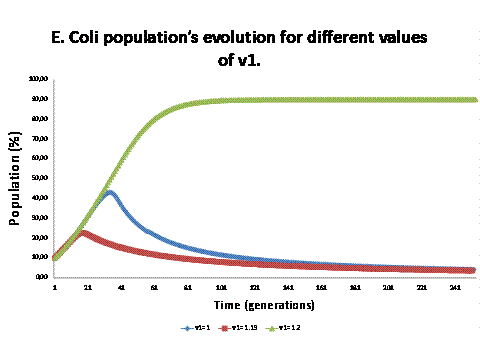Graph 1. Note the very strong step effect between the values of v1 1.19 and 1.20, and the fact that population does not collapse immediately, due to the initial HSL concentration.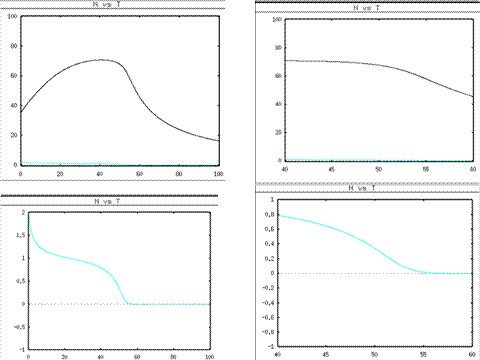Graph 2. In black, the curve of population density (N) against time; in blue, HSL concentration against time, there is a very strong correlation between these two curves.

In the same way, we fixed the value of v1 to 1 and studied the behavior of the population depending of its initial density. We can see (picture3) that for Ninitial=39, the population grows and stabilizes around 80%, when for Ninitial=38, this population density collapses. We still notice that the bacterial density, few instants after the beginning of the simulation, grows in a similar way to what happens for Ninitial=39. This is explained by the non-nul initial concentration of HSL which maintain the population even if the quorum sensing isn't sufficient to produce it during some time (see graph for the parallel between concentration in bacteria and HSL). We chose to give a non-null starting value to the HSL concentration because some is added in the initial growth conditions and, if some bacteria escape in the river, which is similar to a dilution, their intracellular rate of HSL won't be null. However, we can emphasize an important step effect and a bistability phenomenon.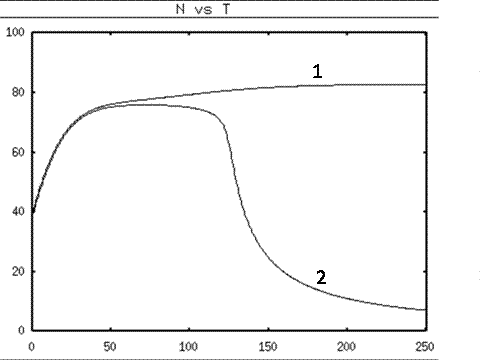Picture 3. That's the step effect which relates to initial cell density. The first curve is of an initial density of 39% and the second has an initial density of 38%. In both situations, populations do not immediately collapse.

This step effect is studied once more in the next graph (graph3) which shows the evolution of the minimal relative transcription speed of the antidote plasmid (v1) such that the population is stable, depending on the initial population density. We logically see the decrease of the v1 value when the initial density increases, but more unexpected, we notice a limit value of v1 (=0.985) such that, no matter how big the initial population is, this population finally collapses. This emphasizes the great importance not to use a too small value of RBS for this plasmid, to avoid the collapse of the bacterial population in the reactor.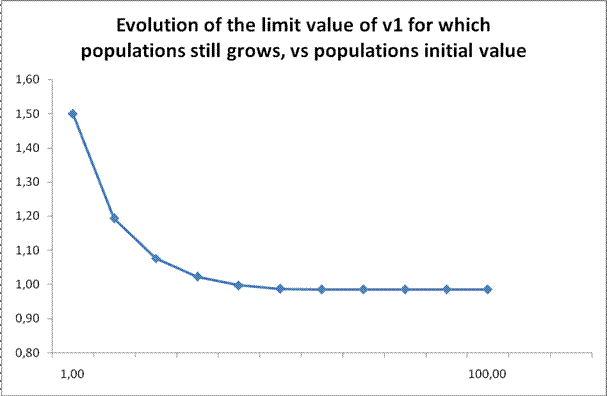Graph 3. At the beginning, a small increase in initial density causes the needed value of v1 to rapidly decrease, then the curve stabilizes and is eventually constant at v1=0,985, for population density value of 60 and more.

Then, using the data computed by the auto software, we draw the stability graph for N (population density, in % of the maximal value in reactor; this symbol will be used in all this section) for different values of v1 (graph4). We see here two disjoint stable curves; the first one, which is a constant equal to zero is the only one for v1<0.985, as expected, and continues for any value of v1. This is explained by the fact that, whatever the value of v1, it's always possible to find a Ninitial such that the population collapses. The second curve only starts at v1=0.985, and the equilibrium value of N increases with v1. This means that, whatever the initial value of the population density, if it's bigger that the step value, the equilibrium value of N will be the same, for a given v1.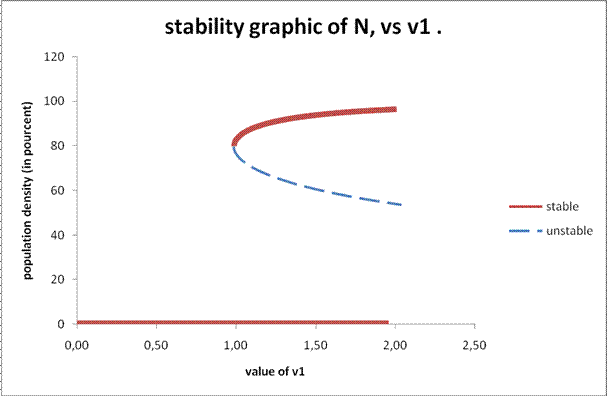Graph 4. Stability graph of N, vs v1. Note the presence of two stable curves for a same value of v1: that's bistability.

Finally we did a little simulation of a real situation, with a population in the reactor initially equal to 100% and a dilution factor of ten, at t=10. The first graph (picture4) is quite clear: during the dilution, the density falls below the critical value, and the population then self-destructs. Zooming on different variables for the population (picture5) we notice the decrease in "growth" proteins (HSL, ParD, LuxR-HSL) and the increase in « destructive » proteins (HSLase, ParE), from the dilution time through a compensation point around 17, in which values are inverted which is the point of no return for the population and until there are no more growth proteins. The system chosen for the programmed cell death thus seems to work perfectly well.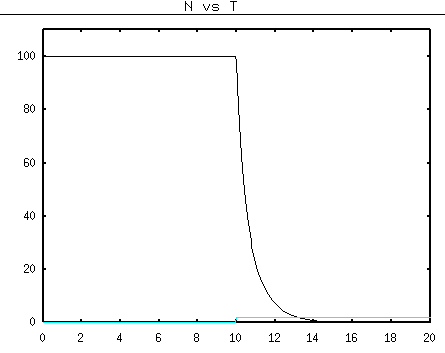Picture 4. Population evolution, before and after the dilution step at t=10. See that, as wanted, the population collapses just after the dilution step, and that it doesn't grow again.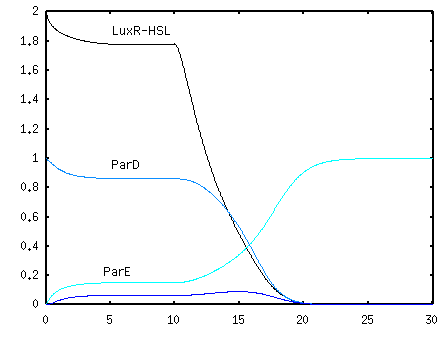Picture 5. Change of some intra-cellular compounds before and after a dilution step at t=10. Note the inversion of the values of ParD and ParE, and the collapse of the activation complex LuxR-HSL.Bacteria planned death system Extra-Scientific issues